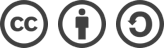## SDUT 3100 动态规划？（动态规划）解题报告

2016-02-16 965 次浏览

## 题面

Time Limit: 1000ms Memory limit: 65536K

1. 这部分元素的下标应满足st，st+5, st+52 , st+53, … , st+5x (0 <= st < n ,st <= st+5x < n)。
2. 在满足第一条要求的方案中，应选取其累加和最大的一种的方案。

10
1 2 3 4 5 6 7 8 9 10
3
1 -10 2
3
-1 -2 -3

15
2
-1

zmx

## 解题思路

#include <cstdio>
using namespace std;

int n, a;

int Solve(int st);

int main(int argc, char const *argv[]) {
while(~ scanf("%d", &n)) {
for(int i=0; i<n; ++i) {
scanf("%d", &a[i]);
}
int max_sum = Solve(0), sum;
//遍历所有 st，并且应注意 n < 5 的情况
for(int i=1; i<5 && i<n; ++i) {
sum = Solve(i);
if(sum > max_sum)
max_sum = sum;
}
printf("%d\n", max_sum);
}

return 0;
}

//求最大连续子序列和
int Solve(int st) {
int max_sum = -100000, this_sum = 0;
for(int i=st; i<n; i+=5) {
this_sum += a[i];
if(this_sum > max_sum)
max_sum = this_sum;
if(this_sum < 0)
this_sum = 0;
}

return max_sum;
}bLue 创作，采用 知识共享署名 3.0，可自由转载、引用，但需署名作者且注明文章出处。